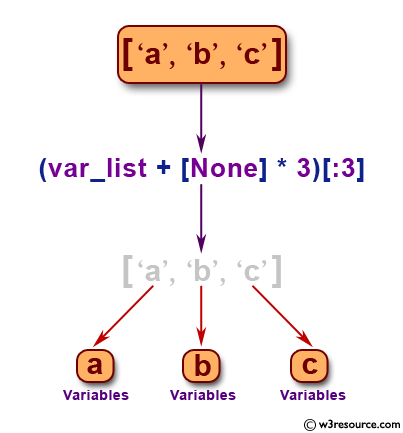﻿ Python: Split a variable length string into variables - w3resource# Python: Split a variable length string into variables

## Python Basic: Exercise-131 with Solution

Write a Python program to split a variable length string into variables.

Sample Solution-1:

Python Code:

``````var_list = ['a', 'b', 'c']
x, y, z = (var_list + [None] * 3)[:3]
print(x, y, z)
var_list = [100, 20.25]
x, y = (var_list + [None] * 2)[:2]
print(x, y)
```
```

Sample Output:

```a b c
100 20.25
```

Pictorial Presentation:## Visualize Python code execution:

The following tool visualize what the computer is doing step-by-step as it executes the said program:

Sample Solution-2:

Python Code:

``````var_list = ['a', 'b', 'c', 'd', 'e']
v, w, x, y, z = var_list
print(v, w, x, y, z)
```
```

Sample Output:

```a b c d e
```

## Visualize Python code execution:

The following tool visualize what the computer is doing step-by-step as it executes the said program:

Python Code Editor:

Have another way to solve this solution? Contribute your code (and comments) through Disqus.

What is the difficulty level of this exercise?

Test your Python skills with w3resource's quiz

﻿

## Python: Tips of the Day

List comprehension:

```>>> m = [x ** 2 for x in range(5)]
>>> m
[0, 1, 4, 9, 16]
```